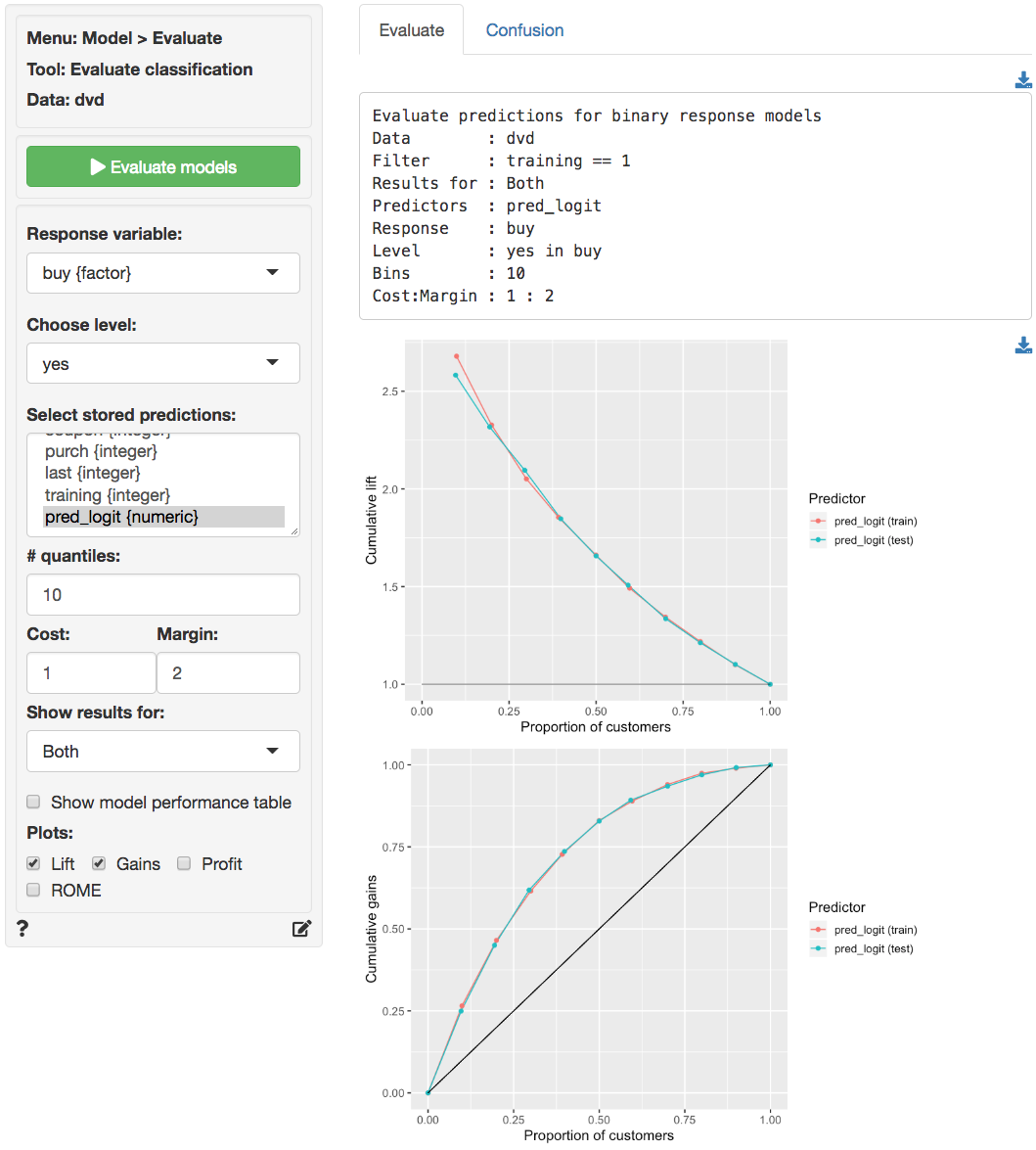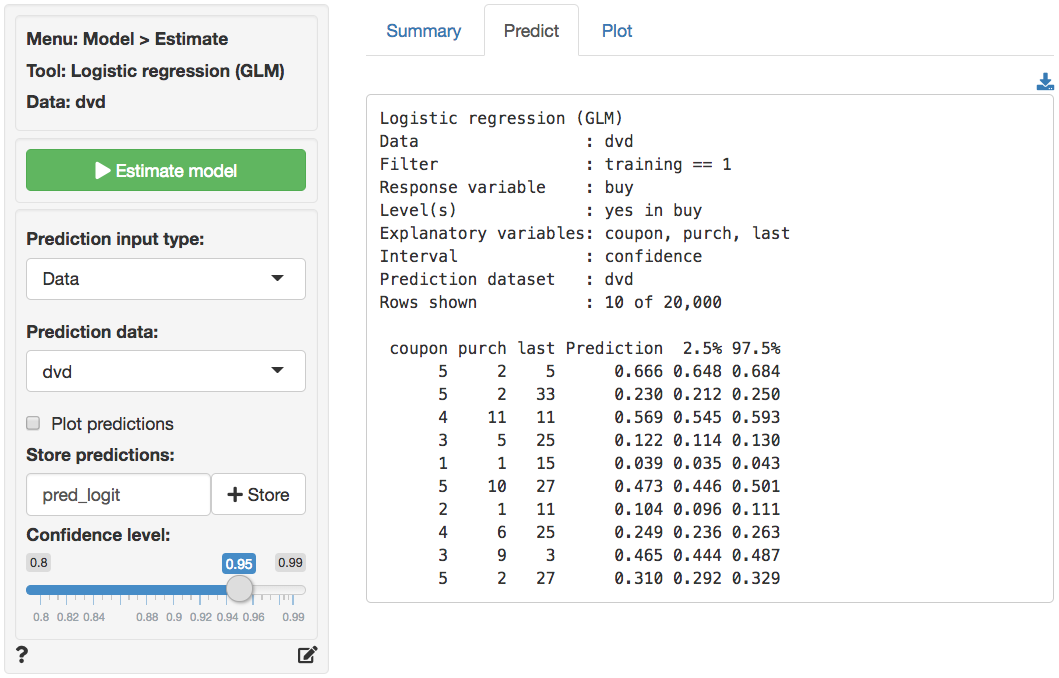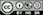Evaluate model performance for (binary) classification

#### Response variable

Select the outcome, or response, variable of interest. This should be a binary variable, either a factor or an integer with two value (i.e., 0 and 1).

#### Choose level

The level in the response variable that is considered a success. For example, a purchase or buyer is equal to “yes”.

#### Predictor

Select one or more variables that can be used to predict the chosen level in the response variable. This could be a variable, an RFM index, or predicted values from a model (e.g., from a logistic regression estimated using Model > Logistic regression (GLM) or a Neural Network estimated using Model > Neural Network).

#### # quantiles

The number of bins to create.

#### Margin & Cost

To use the Profit and ROME (Return on Marketing Expenditures) charts, enter the Margin for each sale and the estimated Cost per contact (e.g., mailing costs or opportunity cost of email or text message). For example, if the margin on a sale is $10 (excluding the contact cost) and the contact cost is$1 enter 10 and 1 in the Margin and Cost input windows.

#### Show results for

If a filter is active (e.g., set in the Data > View tab) generate results for All data, Training data, Test data, or Both training and test data. If no filter is active calculations are applied to all data.

#### Plots

Generate Lift, Gains, Profit, and/or ROME charts.

### Report > Rmd

Add code to Report > Rmd to (re)create the analysis by clicking the icon on the bottom left of your screen or by pressing ALT-enter on your keyboard.

If we create a set of four charts in the Plots tab we can add a title above the group of plots and impose a two-column layout using patchwork as follows:

plot(result, plots = c("lift", "gains", "profit", "rome"), custom = TRUE) %>%
wrap_plots(plot_list, ncol = 2) + plot_annotation(title = "Model evaluation")

The single plot can be customized using ggplot2 commands (see example below)). See Data > Visualize for details.

plot(result, plots = "lift", custom = TRUE) +
labs(caption = "Based on data from ...")

#### Confusion matrix

Predicted probabilities probabilities selected through Predictor are first converted to a class (e.g., a positive or negative outcome) using the values entered in Margin and Cost. It will be profitable to contact a customer if the predicted probability of response exceeds Cost / Margin. For example, if the break-even response rate is 0.1 and the predicted probability of response is 0.25 that customer will be assigned the label Positive. If, on the other hand, the predicted probability does not exceed the break-even response rate a customer will be assigned a Negative label.

Once each prediction has been converted to a class label (i.e., Positive or Negative) the result is compared to the values of the response variable. The following key measures are shown in the generated table for each predictor.

Label Description
TP (True Positive) Number of cases where the positive prediction matches the positive outcome in the data
FP (False Positive) Number of cases with a positive prediction but a negative outcome in the data
TN (True Negative) Number of cases where the negative prediction matches the negative outcome in the data
FN (False Negative) Number of cases with a negative prediction but a positive outcome in the data
total Total number of cases (i.e., TP + FP + TN + FN)
TPR (True Positive Rate) Proportion of positive outcomes in the data that received a positive prediction (i.e., TP / (TP + FN)). Also known as sensitivity or recall
TNR (True Negative Rate) Proportion of negative outcomes in the data that received a negative prediction (i.e., TN / (TN + FP)). Also known as specificity
precision Proportion of positive predictions with a positive outcome in the data (i.e., TP / (TP + FP))
F-score The harmonic mean of precision and TPR (sensitivity)
accuracy Proportion of all outcomes that was correctly predicted as either positive or negative (i.e., (TP + TN) / total)
kappa Corrects the accuracy measure for the probability of generating a correct prediction purely by chance
profit Total profitability achieved by targeting all customers with a predicted probability above the break-even response rate
index Index of relative profitability achieved across the selected Predictor variables (maximum is 1)
ROME Return on Marketing Expenditures (ROME) achieved by targeting all customers with a predicted probability above the break-even response rate
contact Proportion of customers to contact, i.e., (TP + FP) / total
AUC Area Under the ROC Curve (AUC). ROC stands for Receiver Operating Characteristic.

### Report > Rmd (confusion)

Add code to Report > Rmd to (re)create the analysis by clicking the icon on the bottom left of your screen or by pressing ALT-enter on your keyboard.

Only kappa, index, ROME, and AUC are plotted by default. It is possible to customize the plotted results through Report > Rmd. To change the plot use, for example:

plot(result, vars = c("precision", "profit", "AUC"))

The plot can be further customized using ggplot2 commands (see example below)). See Data > Visualize for details.

plot(result, vars = c("precision", "profit", "AUC")) +
labs(caption = "Based on data from ...")

## Example

The Lift and Gains charts below show little evidence of overfitting and suggest that targeting approximately 65% of customers would maximize profits.The prediction used in the screen shots above was derived from a logistic regression on the dvd data. The data is available through the Data > Manage tab (i.e., choose Examples from the Load data of type drop-down and press Load). The model was estimated using Model > Logistic regression (GLM). The predictions shown below were generated in the Predict tab.### R-functions

For an overview of related R-functions used by Radiant to evaluate (binary) classification models see Model > Evaluate classification

© Vincent Nijs (2019)